# Time series analysis in Azure Data Explorer

Azure Data Explorer (ADX) performs on-going collection of telemetry data from cloud services or IoT devices. This data can be analyzed for various insights such as monitoring service health, physical production processes, and usage trends. Analysis is done on time series of selected metrics to find a deviation in the pattern compared to its typical baseline pattern. ADX contains native support for creation, manipulation, and analysis of multiple time series. In this topic, learn how Azure Data Explorer is used to create and analyze thousands of time series in seconds, enabling near real-time monitoring solutions and workflows.

## Time series creation

In this section, we'll create a large set of regular time series simply and intuitively using the `make-series` operator, and fill-in missing values as needed. The first step in time series analysis is to partition and transform the original telemetry table to a set of time series. The table usually contains a timestamp column, contextual dimensions, and optional metrics. The dimensions are used to partition the data. The goal is to create thousands of time series per partition at regular time intervals.

The input table demo_make_series1 contains 600K records of arbitrary web service traffic. Use the command below to sample 10 records:

``````demo_make_series1 | take 10
``````

The resulting table contains a timestamp column, three contextual dimensions columns, and no metrics:

TimeStamp BrowserVer OsVer Country/Region
2016-08-25 09:12:35.4020000 Chrome 51.0 Windows 7 United Kingdom
2016-08-25 09:12:41.1120000 Chrome 52.0 Windows 10
2016-08-25 09:12:46.2300000 Chrome 52.0 Windows 7 United Kingdom
2016-08-25 09:12:46.5100000 Chrome 52.0 Windows 10 United Kingdom
2016-08-25 09:12:46.5570000 Chrome 52.0 Windows 10 Republic of Lithuania
2016-08-25 09:12:47.0470000 Chrome 52.0 Windows 8.1 India
2016-08-25 09:12:51.3600000 Chrome 52.0 Windows 10 United Kingdom
2016-08-25 09:12:51.6930000 Chrome 52.0 Windows 7 Netherlands
2016-08-25 09:12:56.4240000 Chrome 52.0 Windows 10 United Kingdom
2016-08-25 09:13:08.7230000 Chrome 52.0 Windows 10 India

Since there are no metrics, we can only build a set of time series representing the traffic count itself, partitioned by OS using the following query:

``````let min_t = toscalar(demo_make_series1 | summarize min(TimeStamp));
let max_t = toscalar(demo_make_series1 | summarize max(TimeStamp));
demo_make_series1
| make-series num=count() default=0 on TimeStamp from min_t to max_t step 1h by OsVer
| render timechart
``````

In the table above, we have three partitions. We can create a separate time series: Windows 10 (red), 7 (blue) and 8.1 (green) for each OS version as seen in the graph: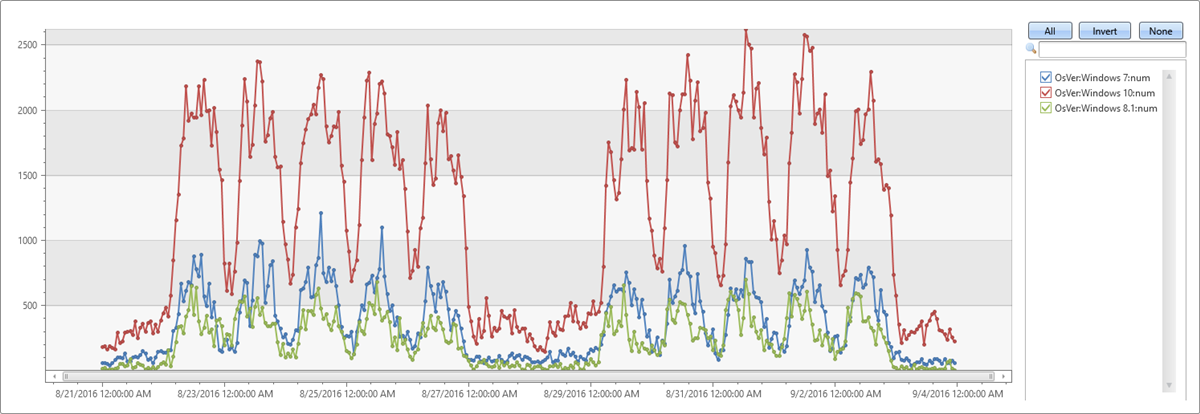## Time series analysis functions

In this section, we'll perform typical series processing functions. Once a set of time series is created, Azure Data Explorer supports a growing list of functions to process and analyze them which can be found in the time series documentation. We will describe a few representative functions for processing and analyzing time series.

### Filtering

Filtering is a common practice in signal processing and useful for time series processing tasks (for example, smooth a noisy signal, change detection).

• There are two generic filtering functions:
• `Extend` the time series set by adding a new moving average series of size 5 bins (named ma_num) to the query:
``````let min_t = toscalar(demo_make_series1 | summarize min(TimeStamp));
let max_t = toscalar(demo_make_series1 | summarize max(TimeStamp));
demo_make_series1
| make-series num=count() default=0 on TimeStamp in range(min_t, max_t, 1h) by OsVer
| extend ma_num=series_fir(num, repeat(1, 5), true, true)
| render timechart
``````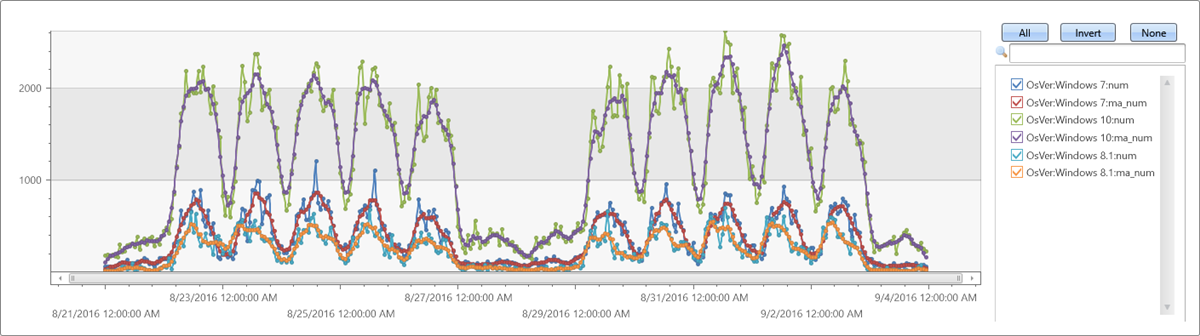### Regression analysis

ADX supports segmented linear regression analysis to estimate the trend of the time series.

• Use series_fit_line() to fit the best line to a time series for general trend detection.
• Use series_fit_2lines() to detect trend changes, relative to the baseline, that are useful in monitoring scenarios.

Example of `series_fit_line()` and `series_fit_2lines()` functions in a time series query:

``````demo_series2
| extend series_fit_2lines(y), series_fit_line(y)
| render linechart with(xcolumn=x)
``````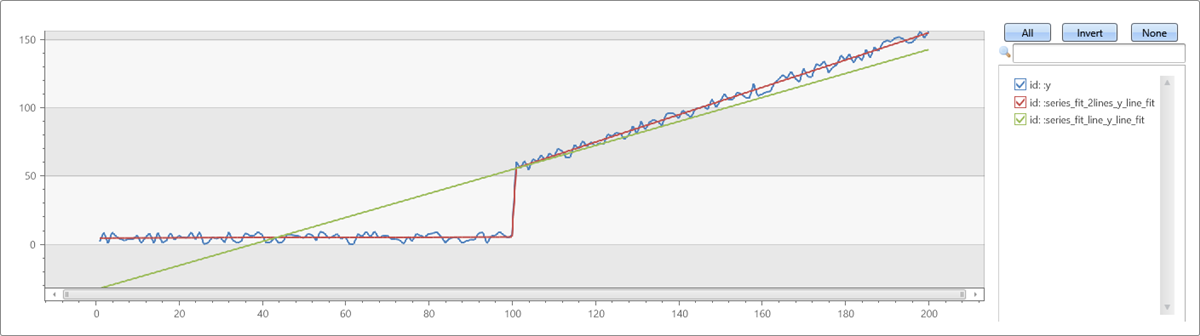• Blue: original time series
• Green: fitted line
• Red: two fitted lines

Note

The function accurately detected the jump (level change) point.

### Seasonality detection

Many metrics follow seasonal (periodic) patterns. User traffic of cloud services usually contains daily and weekly patterns that are highest around the middle of the business day and lowest at night and over the weekend. IoT sensors measure in periodic intervals. Physical measurements such as temperature, pressure, or humidity may also show seasonal behavior.

The following example applies seasonality detection on one month traffic of a web service (2-hour bins):

``````demo_series3
| render timechart
``````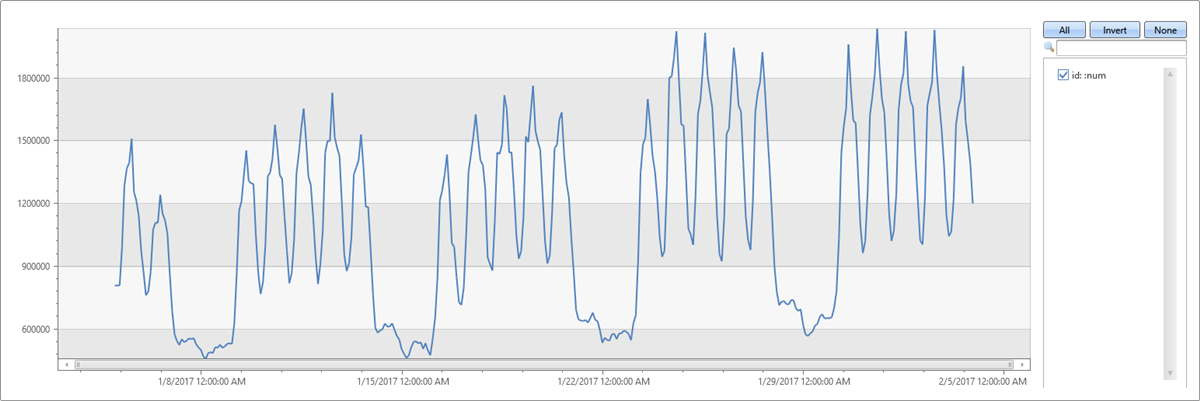Note

It's an anomaly if specific distinct periods don't exist

``````demo_series3
| project (periods, scores) = series_periods_detect(num, 0., 14d/2h, 2) //to detect the periods in the time series
| mv-expand periods, scores
| extend days=2h*todouble(periods)/1d
``````
periods scores days
84 0.820622786055595 7
12 0.764601405803502 1

The function detects daily and weekly seasonality. The daily scores less than the weekly because weekend days are different from weekdays.

### Element-wise functions

Arithmetic and logical operations can be done on a time series. Using series_subtract() we can calculate a residual time series, that is, the difference between original raw metric and a smoothed one, and look for anomalies in the residual signal:

``````let min_t = toscalar(demo_make_series1 | summarize min(TimeStamp));
let max_t = toscalar(demo_make_series1 | summarize max(TimeStamp));
demo_make_series1
| make-series num=count() default=0 on TimeStamp in range(min_t, max_t, 1h) by OsVer
| extend ma_num=series_fir(num, repeat(1, 5), true, true)
| extend residual_num=series_subtract(num, ma_num) //to calculate residual time series
| where OsVer == "Windows 10"   // filter on Win 10 to visualize a cleaner chart
| render timechart
``````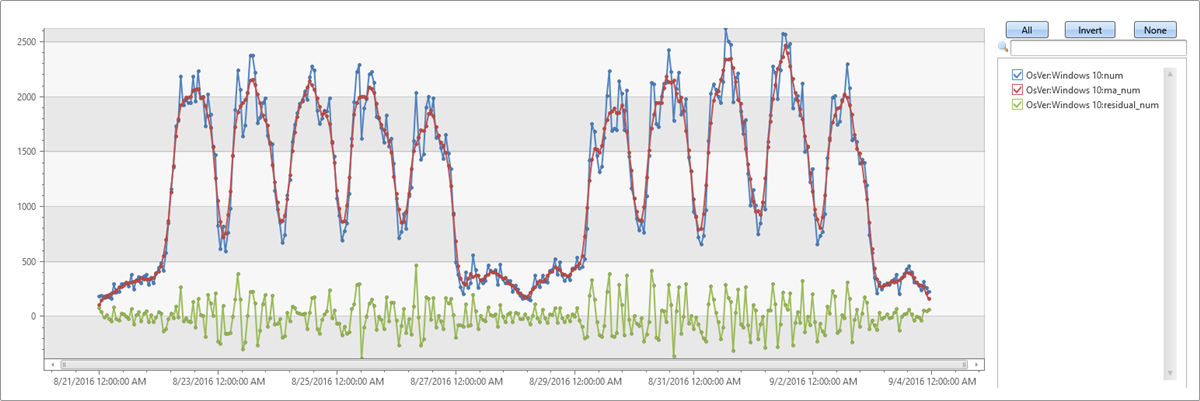• Blue: original time series
• Red: smoothed time series
• Green: residual time series

## Time series workflow at scale

The example below shows how these functions can run at scale on thousands of time series in seconds for anomaly detection. To see a few sample telemetry records of a DB service's read count metric over four days run the following query:

``````demo_many_series1
| take 4
``````
2016-09-11 21:00:00.0000000 Loc 9 5117853934049630089 262 0
2016-09-11 21:00:00.0000000 Loc 9 5117853934049630089 241 0
2016-09-11 21:00:00.0000000 Loc 9 -865998331941149874 262 279862
2016-09-11 21:00:00.0000000 Loc 9 371921734563783410 255 0

And simple statistics:

``````demo_many_series1
| summarize num=count(), min_t=min(TIMESTAMP), max_t=max(TIMESTAMP)
``````
num min_t max_t
2177472 2016-09-08 00:00:00.0000000 2016-09-11 23:00:00.0000000

Building a time series in 1-hour bins of the read metric (total four days * 24 hours = 96 points), results in normal pattern fluctuation:

``````let min_t = toscalar(demo_many_series1 | summarize min(TIMESTAMP));
let max_t = toscalar(demo_many_series1 | summarize max(TIMESTAMP));
demo_many_series1
| render timechart with(ymin=0)
``````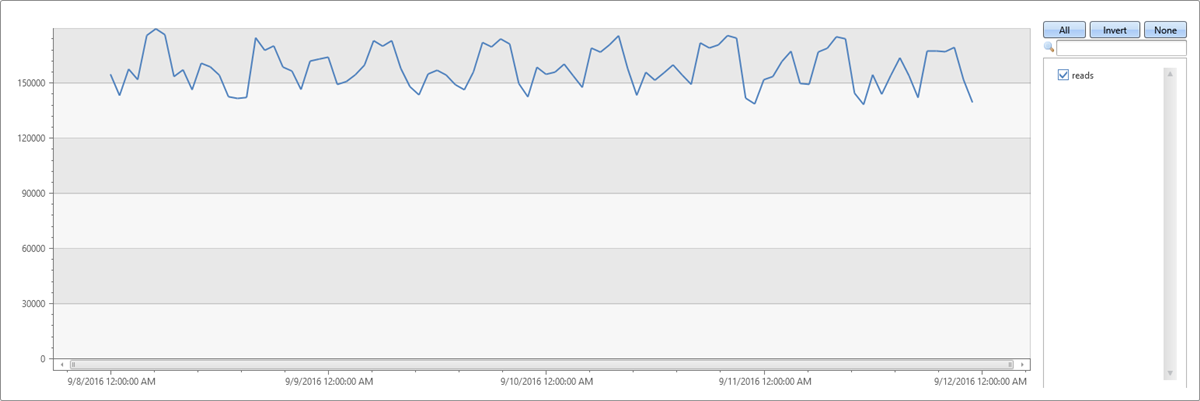The above behavior is misleading, since the single normal time series is aggregated from thousands of different instances that may have abnormal patterns. Therefore, we create a time series per instance. An instance is defined by Loc (location), anonOp (operation), and DB (specific machine).

How many time series can we create?

``````demo_many_series1
| summarize by Loc, Op, DB
| count
``````
Count
18339

Now, we're going to create a set of 18339 time series of the read count metric. We add the `by` clause to the make-series statement, apply linear regression, and select the top two time series that had the most significant decreasing trend:

``````let min_t = toscalar(demo_many_series1 | summarize min(TIMESTAMP));
let max_t = toscalar(demo_many_series1 | summarize max(TIMESTAMP));
demo_many_series1
| make-series reads=avg(DataRead) on TIMESTAMP in range(min_t, max_t, 1h) by Loc, Op, DB
| extend (rsquare, slope) = series_fit_line(reads)
| top 2 by slope asc
| render timechart with(title='Service Traffic Outage for 2 instances (out of 18339)')
``````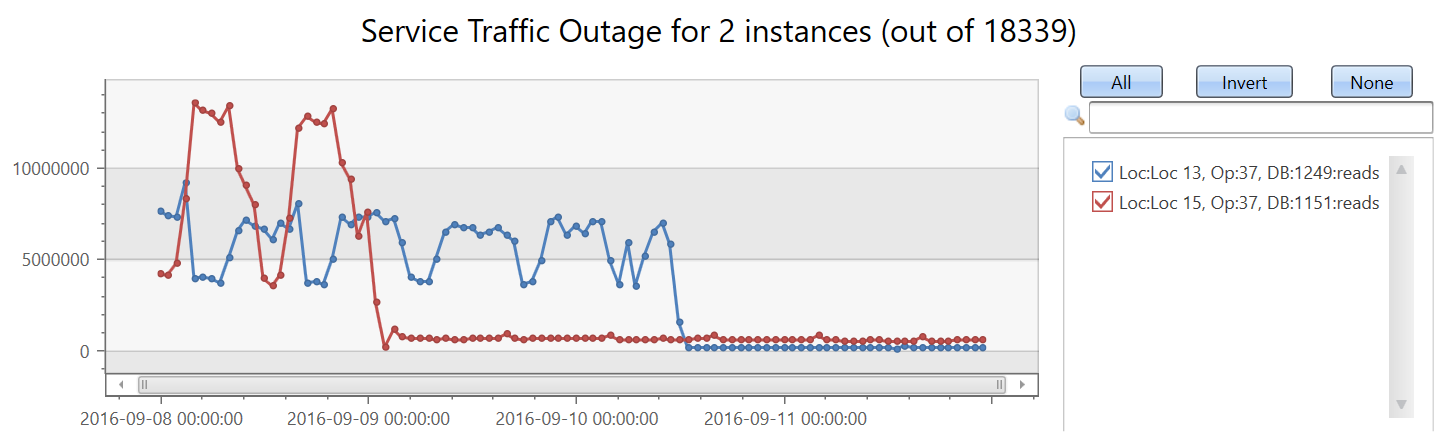Display the instances:

``````let min_t = toscalar(demo_many_series1 | summarize min(TIMESTAMP));
let max_t = toscalar(demo_many_series1 | summarize max(TIMESTAMP));
demo_many_series1
| make-series reads=avg(DataRead) on TIMESTAMP in range(min_t, max_t, 1h) by Loc, Op, DB
| extend (rsquare, slope) = series_fit_line(reads)
| top 2 by slope asc
| project Loc, Op, DB, slope
``````
Loc Op DB slope
Loc 15 37 1151 -102743.910227889
Loc 13 37 1249 -86303.2334644601

In less than two minutes, Azure Data Explorer analyzed close to 20,000 time series and detected two abnormal time series in which the read count suddenly dropped.

These advanced capabilities combined with Azure Data Explorer fast performance supply a unique and powerful solution for time series analysis.